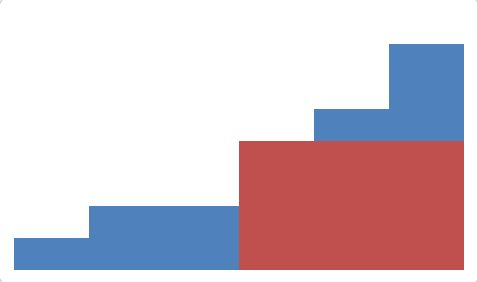# Sofdor Ali and HistogramDRMCSC Programming Contes...
Limits 1s, 512 MB

The Great Sofdor Ali is now interested in competitive programming. Seeing this, his friend and also a renowned scientist Anik Lumba gave him a problem. The problem statement is simple. You have a non-decreasing histogram. You have to find the area of largest rectangle contained within the histogram. For example, in the histogram below, where the 6 bars have heights 1 2 2 4 5 7, the area colored in red is the largest rectangle contained by the histogram. The area of this rectangle is 12.But unfortunately for our great scientist Sofdor Ali, he could not write the solution for this problem. So he asked you to solve the problem.

## Input

First line contains an integer $T$ ($0 < T \le 10$) representing number of test case. Each case starts with an integer $N$ ($0 < N \le 10^5$). Next there are N integers in non-decreasing order representing the heights of columns in the histogram. Height of any column is positive and less than or equal to $10^8$.

For 20 percent of the cases, we have $0 < N \le 100$ and $0 < height \le 100$.

For 50 percent of the cases, we have $0 < N \le 1000$ and $0 < height \le 10000$.

## Output

For each case print the case number and the maximum area of rectangle contained by the histogram.

## Sample

InputOutput
2
5
1 2 3 4 5
8
4 7 22 26 48 78 87 95

Case 1: 9
Case 2: 234MathuDebug

### Submit